# Antiques & Collectibles - November 2nd, 2019

### Auction Details

Choose Your Layout >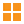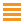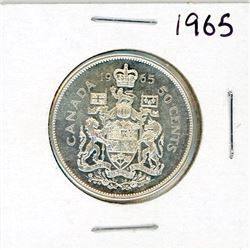701 - FIFTY CENT COIN (CANADA) *1965* (SILVER)
Schmalz Auctions
FIFTY CENT COIN (CANADA) *1965* (SILVER)
Bidding Has Concluded

Sold to tspoon64 for (5.00 + 0.65) x 1 = 5.65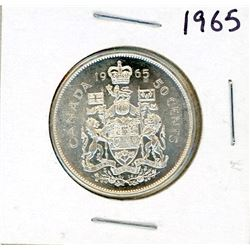702 - FIFTY CENT COIN (CANADA) *1965* (SILVER)
Schmalz Auctions
FIFTY CENT COIN (CANADA) *1965* (SILVER)
Bidding Has Concluded

Sold to oldparts for (7.50 + 0.98) x 1 = 8.48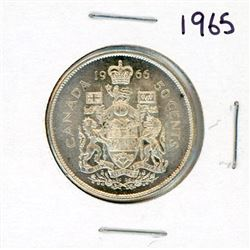703 - FIFTY CENT COIN (CANADA) *1965* (SILVER)
Schmalz Auctions
FIFTY CENT COIN (CANADA) *1965* (SILVER)
Bidding Has Concluded

Sold to oldparts for (7.50 + 0.98) x 1 = 8.48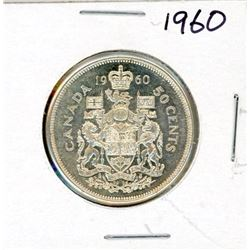704 - FIFTY CENT COIN (CANADA) *1960* (SILVER)
Schmalz Auctions
FIFTY CENT COIN (CANADA) *1960* (SILVER)
Bidding Has Concluded

Sold to RickRain for (10.00 + 1.30) x 1 = 11.30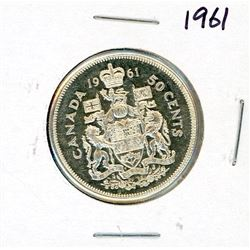705 - FIFTY CENT COIN (CANADA) *1961* (SILVER)
Schmalz Auctions
FIFTY CENT COIN (CANADA) *1961* (SILVER)
Bidding Has Concluded

Sold to RickRain for (10.00 + 1.30) x 1 = 11.30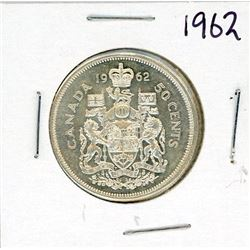706 - FIFTY CENT COIN (CANADA) *1962* (SILVER)
Schmalz Auctions
FIFTY CENT COIN (CANADA) *1962* (SILVER)
Bidding Has Concluded

Sold to oldparts for (7.50 + 0.98) x 1 = 8.48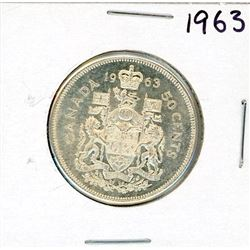707 - FIFTY CENT COIN (CANADA) *1963* (SILVER)
Schmalz Auctions
FIFTY CENT COIN (CANADA) *1963* (SILVER)
Bidding Has Concluded

Sold to oldparts for (7.50 + 0.98) x 1 = 8.48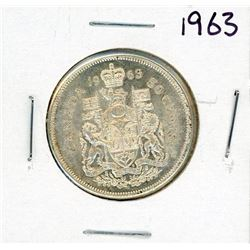708 - FIFTY CENT COIN (CANADA) *1963* (SILVER)
Schmalz Auctions
FIFTY CENT COIN (CANADA) *1963* (SILVER)
Bidding Has Concluded

Sold to oldparts for (7.50 + 0.98) x 1 = 8.48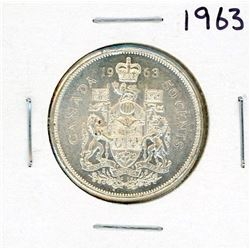709 - FIFTY CENT COIN (CANADA) *1963* (SILVER)
Schmalz Auctions
FIFTY CENT COIN (CANADA) *1963* (SILVER)
Bidding Has Concluded

Sold to oldparts for (7.50 + 0.98) x 1 = 8.48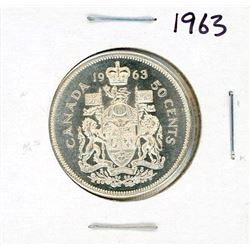710 - FIFTY CENT COIN (CANADA) *1963* (SILVER)
Schmalz Auctions
FIFTY CENT COIN (CANADA) *1963* (SILVER)
Bidding Has Concluded

Sold to oldparts for (7.50 + 0.98) x 1 = 8.48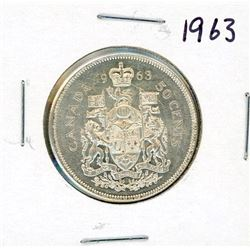711 - FIFTY CENT COIN (CANADA) *1963* (SILVER)
Schmalz Auctions
FIFTY CENT COIN (CANADA) *1963* (SILVER)
Bidding Has Concluded

Sold to oldparts for (7.50 + 0.98) x 1 = 8.48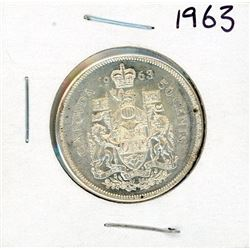712 - FIFTY CENT COIN (CANADA) *1963* (SILVER)
Schmalz Auctions
FIFTY CENT COIN (CANADA) *1963* (SILVER)
Bidding Has Concluded

Sold to oldparts for (7.50 + 0.98) x 1 = 8.48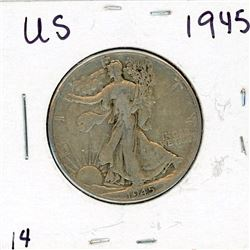713 - FIFTY CENT COIN (USA) *1945* (SILVER)
Schmalz Auctions
FIFTY CENT COIN (USA) *1945* (SILVER)
Bidding Has Concluded

Sold to jdalet for (10.00 + 1.30) x 1 = 11.30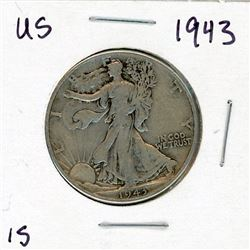714 - FIFTY CENT COIN (USA) *1943* (SILVER)
Schmalz Auctions
FIFTY CENT COIN (USA) *1943* (SILVER)
Bidding Has Concluded

Sold to EJsnow for (12.50 + 1.63) x 1 = 14.13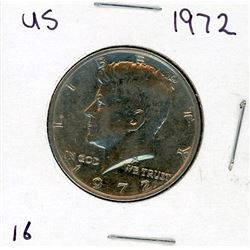715 - FIFTY CENT COIN (USA) *1972*
Schmalz Auctions
FIFTY CENT COIN (USA) *1972*
Bidding Has Concluded

Sold to floor for (5.00 + 0.65) x 1 = 5.65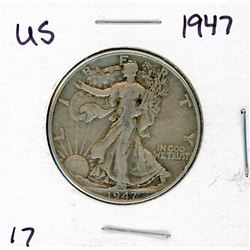716 - FIFTY CENT COIN (USA) *1947* (SILVER)
Schmalz Auctions
FIFTY CENT COIN (USA) *1947* (SILVER)
Bidding Has Concluded

Sold to jdalet for (10.00 + 1.30) x 1 = 11.30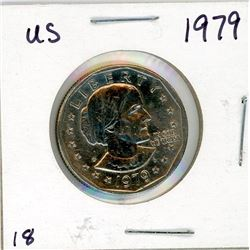717 - ONE DOLLAR COIN (USA) *1979*
Schmalz Auctions
ONE DOLLAR COIN (USA) *1979*
Bidding Has Concluded

Sold to floor for (5.00 + 0.65) x 1 = 5.65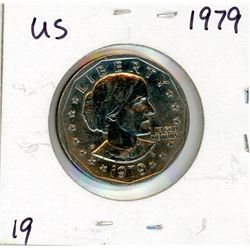718 - ONE DOLLAR COIN (USA) *1979*
Schmalz Auctions
ONE DOLLAR COIN (USA) *1979*
Bidding Has Concluded

Sold to floor for (5.00 + 0.65) x 1 = 5.65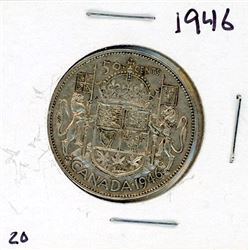719 - FIFTY CENT COIN (CANADA) *1946* (SILVER)
Schmalz Auctions
FIFTY CENT COIN (CANADA) *1946* (SILVER)
Bidding Has Concluded

Sold to Kingrat for (12.50 + 1.63) x 1 = 14.13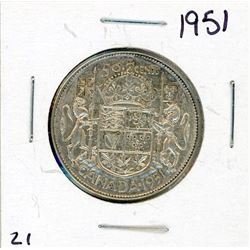720 - FIFTY CENT COIN (CANADA) *1951* (SILVER)
Schmalz Auctions
FIFTY CENT COIN (CANADA) *1951* (SILVER)
Bidding Has Concluded

Sold to Kingrat for (10.00 + 1.30) x 1 = 11.30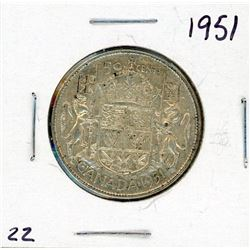721 - FIFTY CENT COIN (CANADA) *1951* (SILVER)
Schmalz Auctions
FIFTY CENT COIN (CANADA) *1951* (SILVER)
Bidding Has Concluded

Sold to oldparts for (7.50 + 0.98) x 1 = 8.48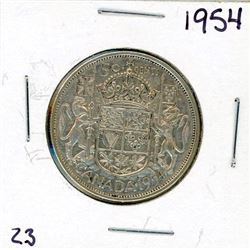722 - FIFTY CENT COIN (CANADA) *1954* (SILVER)
Schmalz Auctions
FIFTY CENT COIN (CANADA) *1954* (SILVER)
Bidding Has Concluded

Sold to Kingrat for (10.00 + 1.30) x 1 = 11.30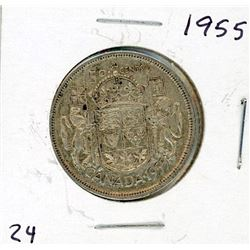723 - FIFTY CENT COIN (CANADA) *1955* (SILVER)
Schmalz Auctions
FIFTY CENT COIN (CANADA) *1955* (SILVER)
Bidding Has Concluded

Sold to Kingrat for (12.50 + 1.63) x 1 = 14.13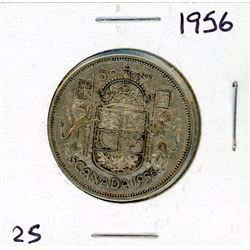724 - FIFTY CENT COIN (CANADA) *1956* (SILVER)
Schmalz Auctions
FIFTY CENT COIN (CANADA) *1956* (SILVER)
Bidding Has Concluded

Sold to floor for (12.50 + 1.63) x 1 = 14.13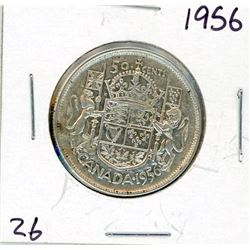725 - FIFTY CENT COIN (CANADA) *1956* (SILVER)
Schmalz Auctions
FIFTY CENT COIN (CANADA) *1956* (SILVER)
Bidding Has Concluded

Sold to Kingrat for (15.00 + 1.95) x 1 = 16.95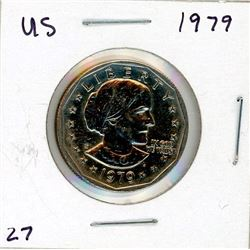726 - ONE DOLLAR COIN (USA) *1979*
Schmalz Auctions
ONE DOLLAR COIN (USA) *1979*
Bidding Has Concluded

Sold to floor for (5.00 + 0.65) x 1 = 5.65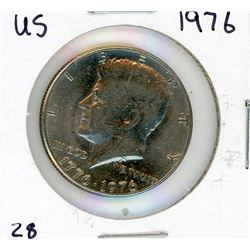727 - FIFTY CENT COIN (USA) *1976*
Schmalz Auctions
FIFTY CENT COIN (USA) *1976*
Bidding Has Concluded

Sold to floor for (7.50 + 0.98) x 1 = 8.48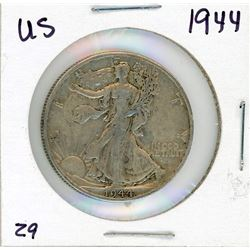728 - FIFTY CENT COIN (USA) *1944* (SILVER)
Schmalz Auctions
FIFTY CENT COIN (USA) *1944* (SILVER)
Bidding Has Concluded

Sold to jdalet for (20.00 + 2.60) x 1 = 22.60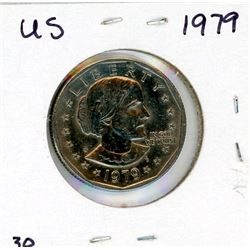729 - ONE DOLLAR COIN (USA) *1979*
Schmalz Auctions
ONE DOLLAR COIN (USA) *1979*
Bidding Has Concluded

Sold to floor for (5.00 + 0.65) x 1 = 5.65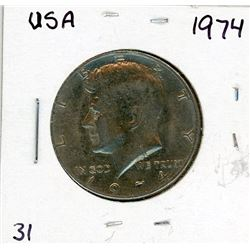730 - FIFTY CENT COIN (USA) *1974*
Schmalz Auctions
FIFTY CENT COIN (USA) *1974*
Bidding Has Concluded

Sold to floor for (7.50 + 0.98) x 1 = 8.48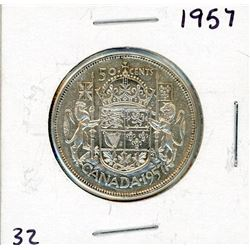731 - FIFTY CENT COIN (CANADA) *1957* (SILVER)
Schmalz Auctions
FIFTY CENT COIN (CANADA) *1957* (SILVER)
Bidding Has Concluded

Sold to Kingrat for (12.50 + 1.63) x 1 = 14.13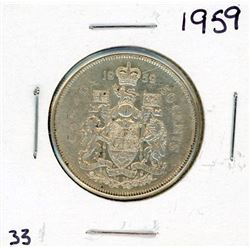732 - FIFTY CENT COIN (CANADA) *1959* (SILVER)
Schmalz Auctions
FIFTY CENT COIN (CANADA) *1959* (SILVER)
Bidding Has Concluded

Sold to oldparts for (10.00 + 1.30) x 1 = 11.30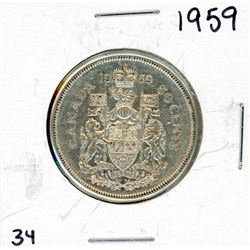733 - FIFTY CENT COIN (CANADA) *1959* (SILVER)
Schmalz Auctions
FIFTY CENT COIN (CANADA) *1959* (SILVER)
Bidding Has Concluded

Sold to Kingrat for (10.00 + 1.30) x 1 = 11.30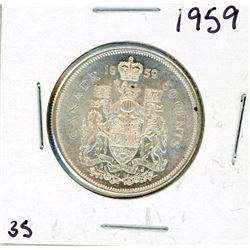734 - FIFTY CENT COIN (CANADA) *1959* (SILVER)
Schmalz Auctions
FIFTY CENT COIN (CANADA) *1959* (SILVER)
Bidding Has Concluded

Sold to Kingrat for (10.00 + 1.30) x 1 = 11.30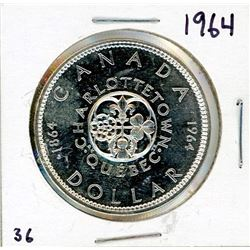735 - ONE DOLLAR COIN (CANADA) *1964* (SILVER)
Schmalz Auctions
ONE DOLLAR COIN (CANADA) *1964* (SILVER)
Bidding Has Concluded

Sold to Argillite for (25.00 + 3.25) x 1 = 28.25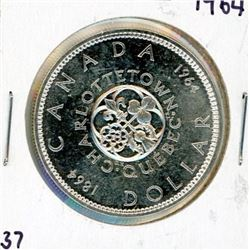736 - ONE DOLLAR COIN (CANADA) *1964* (SILVER)
Schmalz Auctions
ONE DOLLAR COIN (CANADA) *1964* (SILVER)
Bidding Has Concluded

Sold to floor for (17.50 + 2.28) x 1 = 19.78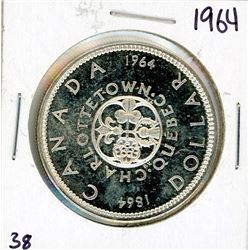737 - ONE DOLLAR COIN (CANADA) *1964* (SILVER)
Schmalz Auctions
ONE DOLLAR COIN (CANADA) *1964* (SILVER)
Bidding Has Concluded

Sold to Kingrat for (17.50 + 2.28) x 1 = 19.78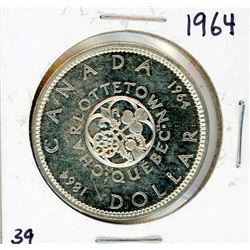738 - ONE DOLLAR COIN (CANADA) *1964* (SILVER)
Schmalz Auctions
ONE DOLLAR COIN (CANADA) *1964* (SILVER)
Bidding Has Concluded

Sold to floor for (15.00 + 1.95) x 1 = 16.95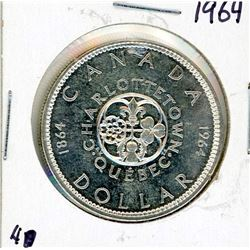739 - ONE DOLLAR COIN (CANADA) *1964* (SILVER)
Schmalz Auctions
ONE DOLLAR COIN (CANADA) *1964* (SILVER)
Bidding Has Concluded

Sold to floor for (15.00 + 1.95) x 1 = 16.95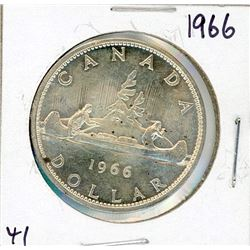740 - ONE DOLLAR COIN (CANADA) *1966* (SILVER)
Schmalz Auctions
ONE DOLLAR COIN (CANADA) *1966* (SILVER)
Bidding Has Concluded

Sold to Kingrat for (17.50 + 2.28) x 1 = 19.78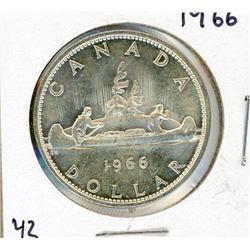741 - ONE DOLLAR COIN (CANADA) *1966* (SILVER)
Schmalz Auctions
ONE DOLLAR COIN (CANADA) *1966* (SILVER)
Bidding Has Concluded

Sold to floor for (15.00 + 1.95) x 1 = 16.95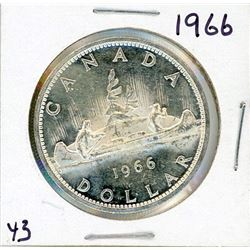742 - ONE DOLLAR COIN (CANADA) *1966* (SILVER)
Schmalz Auctions
ONE DOLLAR COIN (CANADA) *1966* (SILVER)
Bidding Has Concluded

Sold to RickRain for (15.00 + 1.95) x 1 = 16.95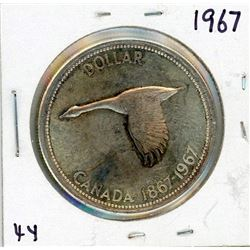743 - ONE DOLLAR COIN (CANADA) *1967* (SILVER)
Schmalz Auctions
ONE DOLLAR COIN (CANADA) *1967* (SILVER)
Bidding Has Concluded

Sold to Kingrat for (17.50 + 2.28) x 1 = 19.78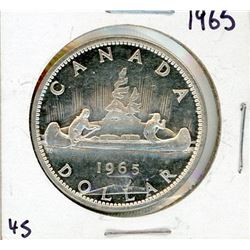744 - ONE DOLLAR COIN (CANADA) *1965* (SILVER)
Schmalz Auctions
ONE DOLLAR COIN (CANADA) *1965* (SILVER)
Bidding Has Concluded

Sold to Kingrat for (22.50 + 2.93) x 1 = 25.43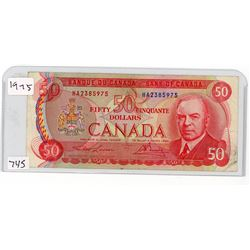745 - FIFTY DOLLAR BILL (CANADA) *1975*
Schmalz Auctions
Bidding Has Concluded

Sold to Kingrat for (60.00 + 7.80) x 1 = 67.80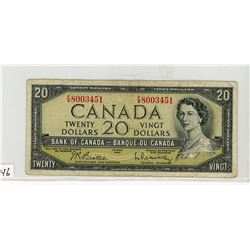746 - TWENTY DOLLAR BILL (CANADA) *1954*
Schmalz Auctions
Bidding Has Concluded

Sold to kingarvid for (30.00 + 3.90) x 1 = 33.90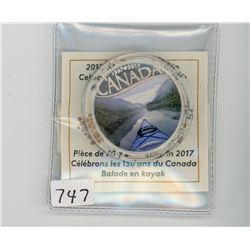747 - TEN DOLLAR COIN (CANADA) *2017* (FINE SILVER) *CELEBRATE CANADA'S 150TH ANNIVERSARY*
Schmalz Auctions
Bidding Has Concluded

Sold to floor for (25.00 + 3.25) x 1 = 28.25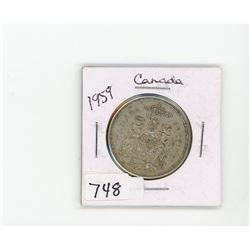748 - FIFTY CENT COIN (CANADA) *1959* (SILVER)
Schmalz Auctions
FIFTY CENT COIN (CANADA) *1959* (SILVER)
Bidding Has Concluded

Sold to floor for (10.00 + 1.30) x 1 = 11.30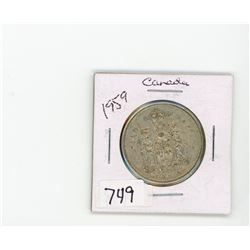749 - FIFTY CENT COIN (CANADA) *1959* (SILVER)
Schmalz Auctions
FIFTY CENT COIN (CANADA) *1959* (SILVER)
Bidding Has Concluded

Sold to oldparts for (7.50 + 0.98) x 1 = 8.48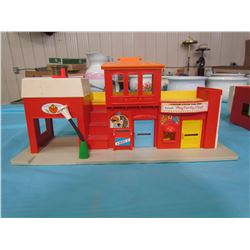750 - THREE ASSORTED FISHER PRICE BUILDINGS (SOME DAMAGE)
Schmalz Auctions
THREE ASSORTED FISHER PRICE BUILDINGS (SOME DAMAGE)
Bidding Has Concluded

Sold to floor for (2.50 + 0.33) x 1 = 2.83